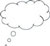# 1 The formal division algorithm

The formal division algorithm below describes very explicitly and formally what division is:

Given any strictly positive integer d (divisor) and any integer a, there exist unique integers q (quotient) and r (remainder) such that

a = qd + r

and

0 < r < d

A strictly positive integer is an integer that is greater than 0.

The description of division given by the two conditions a = qd + r and 0 < r < d is operational. This means that the two conditions give a very explicit way of testing whether or not q is the quotient and r the remainder when the first number (a) is divided by the second (d).

However, these two conditions are not procedural, which means that they do not provide a method for actually finding the quotient and remainder (Lady, 2000). Knowing that 45 is equal to some number multiplied by six, plus some other number, does not in itself give you the procedures to find out what those numbers are.

The formal division algorithm leans towards finding the number that you must multiply the quotient by in order to find a number that is very close to the number a. This is important to note, because if a student’s only secure conception of division is that of sharing (e.g. if I share 45 sweets equally between 6 children how many do they get each? How many are left over?), or grouping (e.g. how many groups of 6 can I make out of 45? How many are left over?), then they will have problems understanding the formal algorithm.

Encouraging your students to think deeply about division is important, and they are much more likely to understand the division algorithm if they have thought about the ideas behind it in a context meaningful to them which they can then express for themselves.Pause for thought Think about how you have taught division in the past. How do you naturally talk about division? For example, if one of your students is having trouble working out 24 ÷ 6, what first comes to your mind? Is it the inverse of multiplication (‘Think about your six times table – how many sixes make 24?’), grouping (‘How many groups of six could you make out of 24?’) or something else?Have you talked to your students about what is the same and what is different about these various ways of talking about division? How could these different ways of talking about division confuse your students?

What you can learn in this unit

2 Being able to see what is happening in division